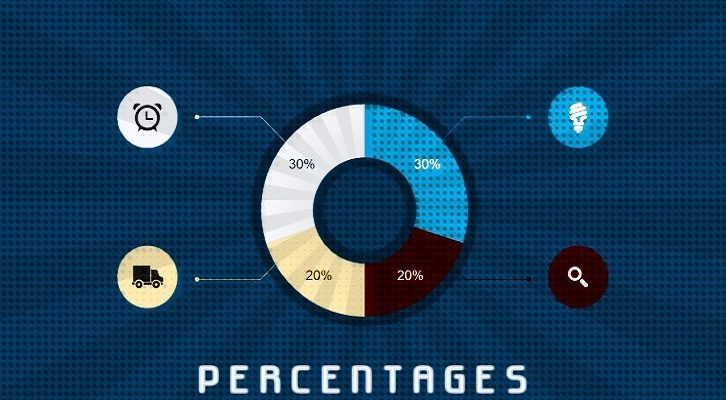# Percentages – Questions

1. a is x % of b, b is x% more than a. Find x?

2. A two digit number ab is 60% of x. The two-digit number formed by reversing the digits of ab is 60% more than x. Find x?
3. A is x % more than B. B is y% less than C. If A,B and C are positive and A > C, find the relation between x and y
4. In a class, if 50% of the boys were girls, then there would be 50% more girls than boys. What percentage of the overall class is girls?
5. Class B has 50% more students than class A. Number of girls in class A is equal to number of boys in class B. The percentage of girls is the same in both classes. What percentage of the student group are boys?
6. A, B, C and D share a loot. A gets a% of the total. B gets b% of the remaining (after A has taken his share). C gets c% of the remaining and D gets the rest. D gets a% less than what A gets. B and C get equal amounts. b = 2a.
i) What percentage of what A got did C get?
ii) If the total amount is equal to Rs. 1000, what is the difference between what A got and what D got?